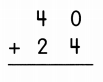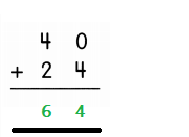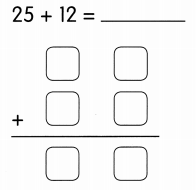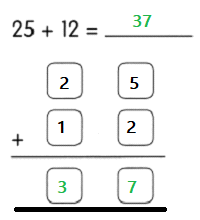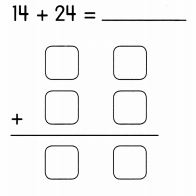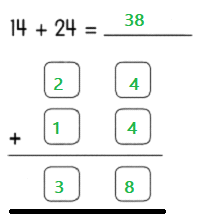Practice the problems of Math in Focus Grade 1 Workbook Answer Key Chapter 13 Practice 1 Addition Without Regrouping to score better marks in the exam.

Example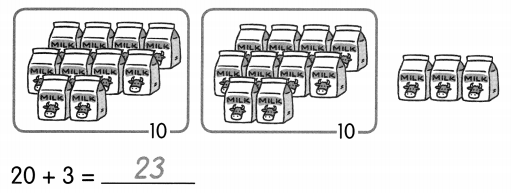Question 1.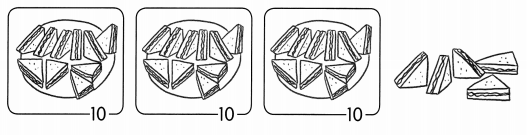30 + 5 = ____
Explanation:
By adding 10 with 10 we get 20 and then by adding 20 with 10 we get 30,
Then adding 30 with 5 we get 35.

Question 2.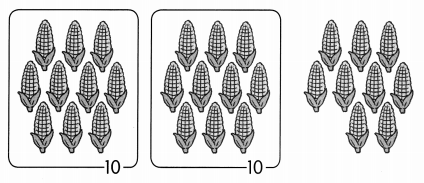20 + 9 = ____
Explanation:
By adding 10 with 10 we get 20,
Then by adding 20 with 9 we get 29.

Question 3.30 + 6 = ____

Explanation:
By adding 10 with 10 we get 20 and then 20 with 10 we get 30,
Then adding 30 with 6 we get 36.

Fill in the missing numbers.

Example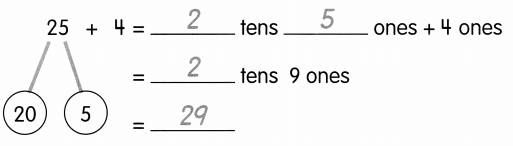Question 4.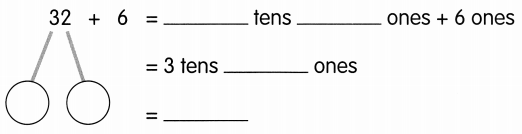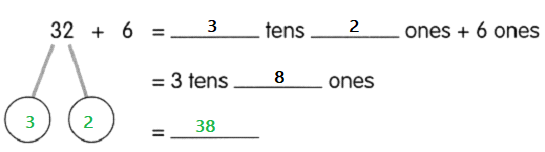Question 5.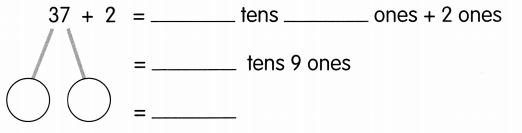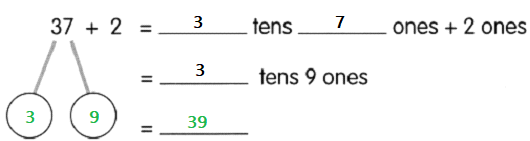Question 6.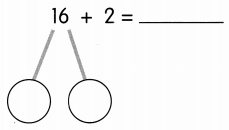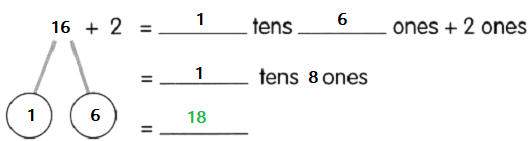Question 7.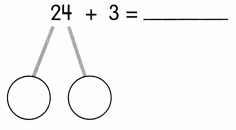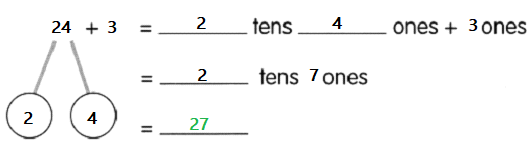Complete each place-value chart Then add.

Example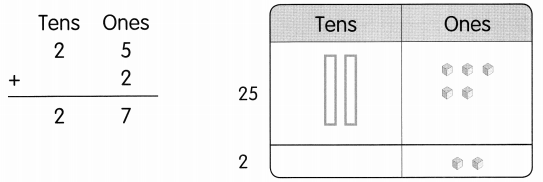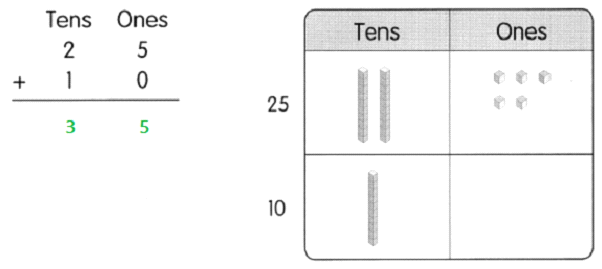Question 8.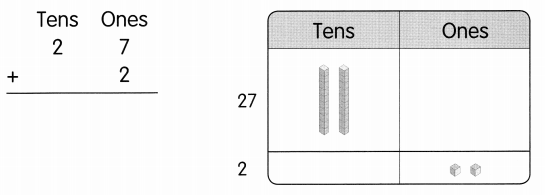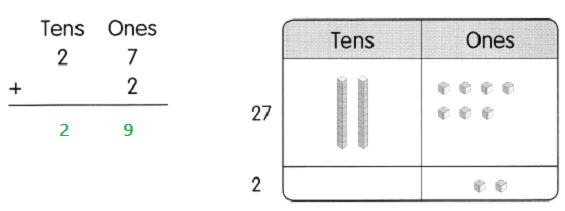Question 9.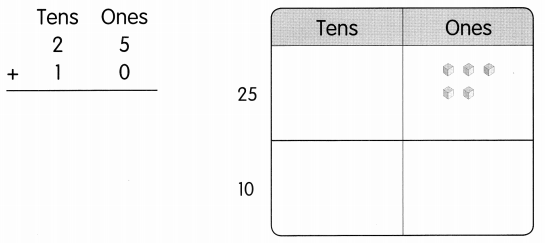Complete the place-value chart. Then add.

Question 10.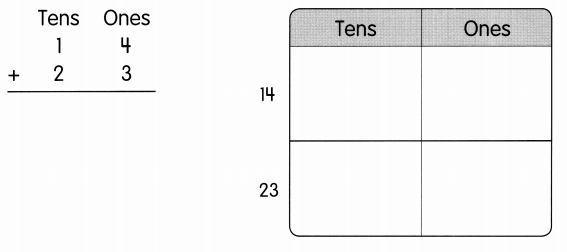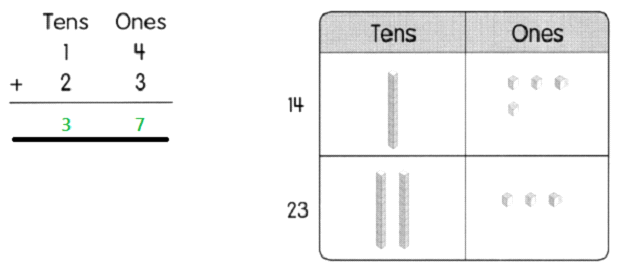Question 11.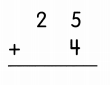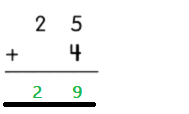Question 12.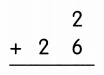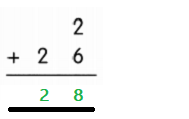Question 13.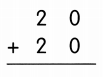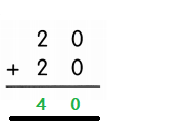Question 14.